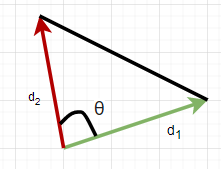# Two airplanes leave an airport at the same time. The velocity of the first airplane is 700 m/h at...

## Question:

Two airplanes leave an airport at the same time. The velocity of the first airplane is 700 m/h at a heading of 52.2 degrees. The velocity of the second is 600 m/h at a heading of 97 degrees. How far apart are they after 3.1 h? Answer in units of m.

## Distance

First, we need to know the distance traveled from the airport by each of the airplanes by using the formula {eq}d = vt{/eq}. After that, we can now find the distance between two airplanes using the cosine law.

Given:

• Velocity of the first plane {eq}v_1 = 700 \rm\ mi/h = 312.93 \rm\ m/s{/eq}
• Velocity of the second plane {eq}v_2 = 600 \rm\ mi/h = 268.22 \rm\ m/s{/eq}
• Angle of the first plane {eq}\theta_1 = 52.2 ^{\circ}{/eq}
• Angle of the second plane {eq}\theta_2 = 97 ^{\circ}{/eq}
• Time {eq}t = 3.1 \rm\ hr = 11,160 \rm\ s{/eq}

The FBD is given belowThe angle {eq}\theta{/eq} is given by

\begin{align} \theta &= \theta_2 - \theta_1\\ \theta &= 97 ^{\circ} - 52.2 ^{\circ}\\ \theta &= \boxed{44.8 ^{\circ}} \end{align}

Next, the distance traveled by each plane is

• Plane 1

\begin{align} d_1 &= v_1 t\\ d_1 &= (312.93 \rm\ m/s)(11,160 \rm\ s)\\ d_1 &= 3492298.8 \rm\ m \end{align}

• Plane 2

\begin{align} d_2 &= v_2 t\\ d_2 &= (268.22 \rm\ m/s)(11,160 \rm\ s)\\ d_2 &= 2993335.2 \rm\ m \end{align}

Now, applying the cosine law to obtain the distance between the two plane

\begin{align} d &= \sqrt{d_1^2 + d_2^2 - 2d_1d_2cos\theta}\\ d &= \sqrt{(3492298.8 \rm\ m)^2 + (2993335.2 \rm\ m)^2 - 2(3492298.8 \rm\ m)(2993335.2 \rm\ m)cos(44.8 ^{\circ})}\\ d &= \boxed{2514167.67 \rm\ m} \end{align}